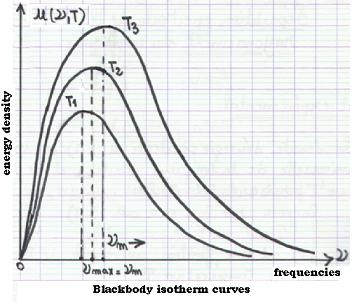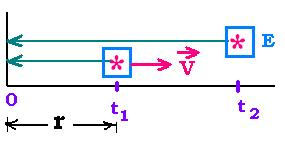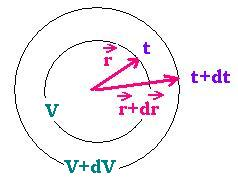Projectile's charge Z Projectile's E (MeV)

Contents

The nucleus

Statistical Mechanics

Related topics

### 1. The experimental resultThe spectral energy density u(λ,T) is an universal function of the wavelength λ and the teperature T as it is the specific spaectral intensity E(λ,T).
With ν = c/λ, we have: u(λ, T) = (ν/c2) u(λ,T)

u(ν,T) is an universla function of the frequency ν and the temperature T.

The main issue, regarding the study of the blackbody rays in the determination of this universal function. The Stefan law is not sufficient to show all the needed blackbody features.

The experimental results have shown that the profile of the enrgies emitted from a blackbody cavity related to monochromatic radiations, for a given temperature, is a "bell curve". The set of all this profiles is known as the blackbody isotherms.

### 2. Wien displacement law

Since λ = c/ν, we have dλ = (c/ν2) dν
The energy of the radiation is written as:
W(λ) = (ν2/c) W(ν) or W(ν) = (λ2/c) W(λ)Now, leT 's consider a signal emitted at the time t1 from an emitter E moving at the velocity v. For the observer, this signal reaches the point 0 at the time t1' = t1 + r/c
If another signal is emited at the time t2 by the emitter, it will reach the point 0 at the time t2' = t2 + (t2 - t1)v/c + r/c.
We have then : t2' - t1' = t2 - t1 + (t2 - t1)v/c = (t2 - t1)( 1 + v/c).
If the signal is a radiation of period t2 - t1; this period measered in the fixed frame 0 is t2' - t1'. Assuming that v << c, forethermore, the frequency of the radiation is 1/(t2' - t1') = 1/(t2' - t1')(1 + v/c) = (1 - v/c)(t2' - t1'). With ν' = 1/(t2' - t1') and ν = 1/(t2 - t1), we have ν' = ν(1 - v/c), known as Doppler effect.
LeT 's write dν = ν' - ν. We have then:
dν/ν = - v/c

We assume that radiation takes place inside a volume V with reflecting (adiabatic) faces that if they contract or expand the compression remains an isentropic process (the entropie S is constant). From the relationship (13.3), if we differentiate S = (4/3) a T3, we find: dS = 0 → dV/V + 3dT/T = 0
dV/V + 3dT/T = 0We consider that the volume V is expanded by dV from r to r+dr. During the time interval dt, between r and r + dr, a point on the cercle of the volume V moved to the cercle of the volume V+dV with a velocity v = dr/dt. The radiation travelled, during the ng the same interval of time dt, the distance r + dr = c dt. We can write :
(r + dr)/c = dr/v. As dr << r, we have then:
dr/r = v/c
With the Doppler effect,V = (4/3)πr3, and from the relationship dV/V + 3dT/T = 0, we have dν/ν = - v/c = - dr/r = - (1/3) dV/V = dT/T
dν/ν = dT/T
Integrating the above equality, we find ν/T = constant, especially at the maximum:

#### νmax/T = constant

Wien's displacement law

### 3. Wien formula

LeT 's consider a frequency interval Δ ν that becomes by an isentropic process,Δ ν'. We can write:
d(Δ ν)/Δ ν = dT/T.

The Stefan formula u = a T4, where u is the energy density per volume unit, gives du/u = d(Δu)/Δu = 4dT/T

The energy density u depends on λ or ν and T. We can write:
du = u(ν,T)dν then Δu = u(ν,T) Δν.
It follows that:
d(Δu)= du(ν,T)Δν + u(ν,T) d(Δν).
Dividing by u(ν,T)Δν , we have:
d(Δu) /u(ν,T)Δν = du(ν,T)/u(ν,T) + d(Δν) Δν
or:
4dT/T = du(ν,T)/u(ν,T) + d(Δν) Δν + dT/T
thus:
du(ν,T)/u(ν,T) + d(Δν) Δν = 3dT/T
Integrated becomes:
du(ν,T) = T3 f(ν,T)
f(ν,T) is a function depending on ν and T.

If we integrate for all the frequencies, we have to find the Stefan law:
U = aT4 = ∫ du(ν,T)dν [ν: 0 → + ∞ ]
We have:
U = aT4 = ∫ du(ν,T)dν = ∫ T3 f(ν,T) dν
or:
aT4 = ∫ T3 f(ν,T) dν = T4 ∫ f(ν,T) dν/T
Hence:
The constant a = ∫ f(ν,T) d(ν/T) and f(ν,T) has to be written as: f(ν,T) = f(ν/T). Then:

u(ν,T) = T3f(ν/T)
That is the Wien formula

But this function will remains unknown.

### 4. Conclusion

In a black body cavity at a temereture T, the frequency of a radiation ν chnage into ν' when the temperature change into T '; but the ratio ν/T = ν'/T ' = Constant.
The specral energy density u(ν,T), or the energy of transported by a radiation of frequency ν at the temerature T, per volume unit becomes u'(ν',T ') and we have, according to the Stefan law:
u(T ') /u(T) = (T '/T)4
And according to the Wien formula, we have:
u(ν',T ')/u(ν,T) = (T '/T)3 f(ν'/T ')/f(ν/T)
Since f(ν'/T ') = f(ν/T), we have:
u(ν',T ')/u(ν,T) = (T '/T)3

Then:
ν' = (T '/T)ν
u(T ') = u(T)(T '/T)4
u(ν',T ') = u(ν,T) (T '/T)3

The set of the isotherm curves of u(ν,T) is build from the single function u(ν,T) by a translation of the abscissa ν to ν' = (T '/T)ν and the ordinate u(ν,T) to u(ν',T ') = u(ν,T) (T '/T)3 simultanously.

We will have the same results with λ = c/ν:
We have: u(λ,T) = (ν2 /C) u(ν,T) = (c/λ2) u(ν,T)
According to Wien formula:
The: u(λ,T) = (c/λ2) T3 f(ν/T) = (c/λ2) T3 f(c/λT)

As we have ν/T = constant; we have also λT = constant. That is:
λ' = (T/T ') λ
Hence: f(c/λT) = f(c/λ'T ') = constant
Thus:
u(λ',T ') = (λ/λ')2 (T '/T)3 u(λ,T)
= (T '/T)2 (T '/T)3 u(λ,T) = (T '/T)5 u(λ,T)

λ' = (T/T ') λ
u(λ',T ') = (T '/T)5 u(λ,T)

The set of the isotherm curves of u(λ,T) is build from the single function u(λ,T) by a translation of the abscissa λ to λ' = (T/T ')λ and the ordinate u(λ,T) to u(λ',T ') = u(ν,T) (T '/T)5 simultanously.Web ScientificSentence